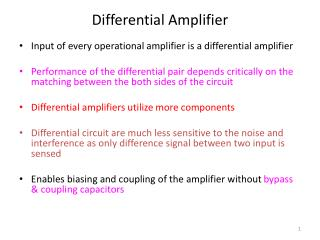DownloadDownload PresentationDifferential Amplifier

# Differential Amplifier

Télécharger la présentation## Differential Amplifier

- - - - - - - - - - - - - - - - - - - - - - - - - - - E N D - - - - - - - - - - - - - - - - - - - - - - - - - - -
##### Presentation Transcript

1. Differential Amplifier • Input of every operational amplifier is a differential amplifier • Performance of the differential pair depends critically on the matching between the both sides of the circuit • Differential amplifiers utilize more components • Differential circuit are much less sensitive to the noise and interference as only difference signal between two input is sensed • Enables biasing and coupling of the amplifier without bypass & coupling capacitors

2. Figure 7.1 The basic MOS differential-pair configuration.

3. Differential Amplifier • Q1& Q2 are two matched transistors • Constant current source (I) provides dc biasing & is an ideal source • RD1=RD2 • It is essential that the MOSFET remain in saturation mode all the time

4. Figure 7.2 The MOS differential pair with a common-mode input voltage vCM. Microelectronic Circuits - Fifth Edition Sedra/Smith

5. Figure 7.3 Circuits for Exercise 7.1. Effects of varying vCM on the operation of the differential pair. Microelectronic Circuits - Fifth Edition Sedra/Smith

6. Figure 7.3(Continued) Microelectronic Circuits - Fifth Edition Sedra/Smith

7. Figure 7.4 The MOS differential pair with a differential input signal vid applied. With vid positive: vGS1>vGS2, iD1>iD2, and vD1<vD2; thus (vD2-vD1) will be positive. With vid negative: vGS1<vGS2, iD1<iD2, and vD1>vD2; thus (vD2-vD1) will be negative. Microelectronic Circuits - Fifth Edition Sedra/Smith

8. Figure 7.5 The MOSFET differential pair for the purpose of deriving the transfer characteristics, iD1 and iD2 versus vid=vG1 – vG2. Microelectronic Circuits - Fifth Edition Sedra/Smith

9. Figure 2.4 Representation of the signal sources v1 and v2 in terms of their differential and common-mode components. Microelectronic Circuits - Fifth Edition Sedra/Smith

10. Figure 7.8 Small-signal analysis of the MOS differential amplifier: The circuit with a common-mode voltage applied to set the dc bias voltage at the gates and with vid applied in a complementary (or balanced) manner.

11. Figure 7.8 Small-signal analysis of the MOS differential amplifier The circuit prepared for small-signal analysis.

12. Figure 7.8 Small-signal analysis of the MOS differential amplifier: An alternative way of looking at the small-signal operation of the circuit.

13. Figure 7.9(a) MOS differential amplifier with ro and RSS taken into account.

14. Figure 7.9 Equivalent circuit for determining the differential gain.

15. Figure 7.10 The MOS differential amplifier with a common-mode input signal vicm.

16. Figure 7.10 Equivalent circuit for determining the common-mode gain (with ro ignored). Each half of the circuit is known as the “common-mode half-circuit.”

17. Figure 7.11 Common-mode gain resulting from a mismatch in the gm values of Q1 and Q2.

18. Assignment # 2 Exercise 7.5 and 7.6 Problem 7.15, 7.16, 7.17 Due date: 20th October 2011